India: +91 9071-449-888, +91 9620-196-773
USA: +1 415-529-4858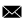info@iqstreamtech.com
Call for Free Demo +91 9071-449-888, +91 9620-196-773, 080-42073645

#### Nearby Locations

Nearby Locations: BTM Layout, Jayanagar, JP Nagar, Koramangala, HSR Layout, Bommanahalli# Best DataScience Training in Bangalore

## Bangalore, Delhi, Mumbai, and Chennai

IQ Stream Technologies is one of the top Data Science with Python and Machine Learning Training institutes in Bangalore with highly experienced and skilled trainers. IQ Stream Technologies Bangalore also offers placement assistance for students who enrolled in Advanced Data Science Training Courses. We offer advanced Data Science with Python and Machine Learning training and advanced tools for better learning, understanding and experience. IQ Stream Technologies also offering Data Science with Python and Machine Learning online training for Bangalore, Delhi, Mumbai, and Chennai students.

## Expert Data Science with Python and Machine Learning Training

Become an Expert in Data Science with Python and Machine Learning with IQ Stream Technologies' advanced Data Science learning programmes. Main highlights of our Data Science with Python and Machine Learning Training in Bangalore (BTM Layout), Delhi, Mumbai, and Chennai are Introduction to Big Data Analytics, What is Data Science?, Data Analytics Lifecycle, Environment Set-Up, Python Crash Course, Data Preprocessing, Introduction to Machine Learning, Data Visualization using Tableau, Regression, Classification, Clustering, Association Rule Learning, Natural Language Processing, many more and Intensive Practical Training, Advanced Learning Materials & Tools, Friendly Classrooms, World class online infrastructure, hands-on labs, real-world tasks etc. IQ Stream Technologies offer beginner, intermediate and advanced lessons for you to become an expert in the area. We will also help you out on understanding the real life approach on Data Science and Machne learning, Preparation of Resume, Mock Interviews and whatever the help you need to leverage your current experience and learning from training to get on to your next move in your career.

IQ Stream Technologies Data Science with Python and Machine Learning HCM Training Institute Location: BTM Layout, Bangalore

Also in: Marathahalli, Hebbal, KR Puram, Whitefield## Course Overview:

 Course Name Data Science Training Venue IQ Stream Technologies Official URL Data Science Training Demo Classes On Demand Training Methodology: 25% Theory & 75% Practical Course Duration 40-45 Hours Class Availability Weekdays & Weekends Demo Training Email ID : info@iqstreamtech.com

## 5 Star Reviews & Ratings

Reviewed By: Tharani Dharan
Reviewed Date: March, 2018
I took Fusion HCM training at IQ Stream Technologies.They have good teaching staff like Mr.Sachin and Bhaskar. The management is very professional and helpful, Affordable fees and quality courses offered.

Reviewed By: Zaki
Reviewed Date: March, 2018
I attended the Oracle Cloud Fusion session in IQ Stream Technologies. Sachin is the Trainer and the Training was good. The trainer explained the fundamental concepts very clearly and showed the roadmap of how to independently explore deeper into details.

Reviewed By: Naresh Bogavaram
Reviewed Date: March, 2018
The best thing i like at IQ stream is the personalized care that the trainers take to make me understand concepts well. The experienced trainers make it easy to learn.

Nearby Locations: BTM Layout, Jayanagar, JP Nagar, Koramangala, HSR Layout, Bommanahalli

## Data Science with Python and Machine Learning Syllabus

### What is Data Science?

What is Data Science?
Structure of Data Science team
Role of a data scientist

### Data Mining and Analytics Lifecycle

Data Lifecycle
Problem Definition
Data Collection
Cleansing Data
Data Exploration
Introduction to SQL
Data Tools
Decision trees
Naive Bayes Classifier
K Means Clustering Modelling errors and resolutions

### Python Crash Course

This module will be based on the expertize of the attendees on Python. Below are some topics:

Overview of Python
Relevance of Python and Data Scince and Machine Learning
Programming style in Python
Objects
List
Tuple
Dictionary
Functions
Lamda Programming
Pandas
Numpy
Datavisualization

### Basics Statistics and Implement using Python

Different types of Data
Mean, Median, Mode
Using Mean, Median and Mode in Python
Variation and Standard Deviation
Probability
Data Distributions
Percentiles
Moments
Covariance and Correlation
Conditional Probability
Bayes' Theorem

### Introduction to Machine Learning

Teaching computer to learn concepts using data - without explicitly programmed
Patterns in data, what does it mean?
Representing reality in models
What is Machine Learning?
Requisites for Machine Learning
Supervised Learning
Unsupervised Learning

### Machine Learning projects - Get Ready

Prepare project objectives
Identify data items
Identify sources of data
Data cleansing
Preparing data for Machine Learning
Supervised machine learning
Naive Bayesian classifiers Unsupervised Learning Methods
Unsupervised machine learning

### Data Visualization using Tableau

Environment Setup
Design Flow
File and Data Types
Terminology
Data Sources
Extracting Data
Custom Data View and Data Joining
Metadata and Data Blending
Tableau Woksheets
Tableau Calucations
Tableau Sorting & Filtering
Tableau Various Charts
Data Compression
Visualization
Reconstruction from Compressed Representation

### Regression

Linear Regression - One variable
Inverse and Transpose
Matrices and Vectors
Linear Regression - Multiple variables
Normal distribution and its applications
Confidence levels and confidence interval
Measure of significance, area of acceptance, area of rejection
P Value, Z scores
Sample, sampling and population
Logistic Regression
Hypothesis and hypothesis testing
Decision Boundary
Infer about population from sample
Regularized Linear Regression
Regularized Logistic Regression

### More Statistics

Multivariate analysis
Interpreting R Square analysis
Selecting attributes for dimensions, independent dimensions
Correlation filters
Low variance filters
Mutual information filters
Principal component analysis

### Neural Networks

Non-linear Hypotheses
Neurons and Brain
Model Representation
Multiclass Classification

Python Training

## Types of Data

Discrete Numerical Data: This is Integer based.
Example: Population of cities

Continuous Numerical Data: Infinite number of possible values ie., it may contain fractions.
Example: Height of a person

Categorical Data: Doesn't have inherent numeric meaning.
Example: Gender, Product category

Ordinal Data: Mixure of Numerical and Categorical
Example: Movie Ratings on a scale of 1-5

## Mean, Median, Mode

Mean: This is average of all the sample values. Sum / Number of samples
Example: 2, 5, 3, 7, 4 , 8, 4, 1, 9
Mean => (2+5+3+7+4+8+4+1+9)/9 = 4.89

Median: The mid value of all the samples sorted
Example: 2, 5, 3, 7, 4 , 8, 4, 1, 9
Sorted: 1,2,3,4,4,5,7,8,9
Median is 4
If the number of sample values are even, then take the average of the two mid values
Median is considered better than Mean when there are outliers in the samples as Mean would be skewed.

Mode: The sample value that has most number of occurances
Example: 2, 5, 3, 7, 4 , 8, 4, 1, 9
Mode: 4 as it is appearing two times where as all other sample values are appearing only once.

## Mean, Median and Mode in Python

Program
import numpy as np
from scipy import stats as st
age = [20, 40, 30, 60, 85, 64, 23, 56, 78, 56, 34, 56, 78, 34, 67, 65]
print ("Mean:",np.mean(age))
print ("Median:", np.median(age))
print ("Mode:", st.mode(age))

Output Mean: 52.875
Median: 56.0
Mode: ModeResult(mode=array(), count=array())

## Histogram

In a set of values, identify the frequency of occuring of each of the values and plot them as a bar graph by each bar representing frequency of each value.
Ex: Below is the list of age of the people attending an event
25, 30, 45, 60, 25, 45, 34, 56, 25, 30, 30, 25, 45, 60, 25
You can make a table of ranges of ages and number of values in each range

Age Range and Frequency
20-29: 5
30-39: 4
40-49: 3
50-59: 1
60-69: 2

If you plot these value on a bar graph, taking age range on X and Frquency on Y, that gives a histogram.

## Program: Plot a Histogram

import numpy as np
import matplotlib.pyplot as plt

#Generate ages instead of hard coding, we will get more meaningful values
#40 is centered values
#5 standard deviation
#1000 number of values
ages = np.random.normal(40, 5, 1000)
plt.hist(ages, 50)
plt.show()

Output: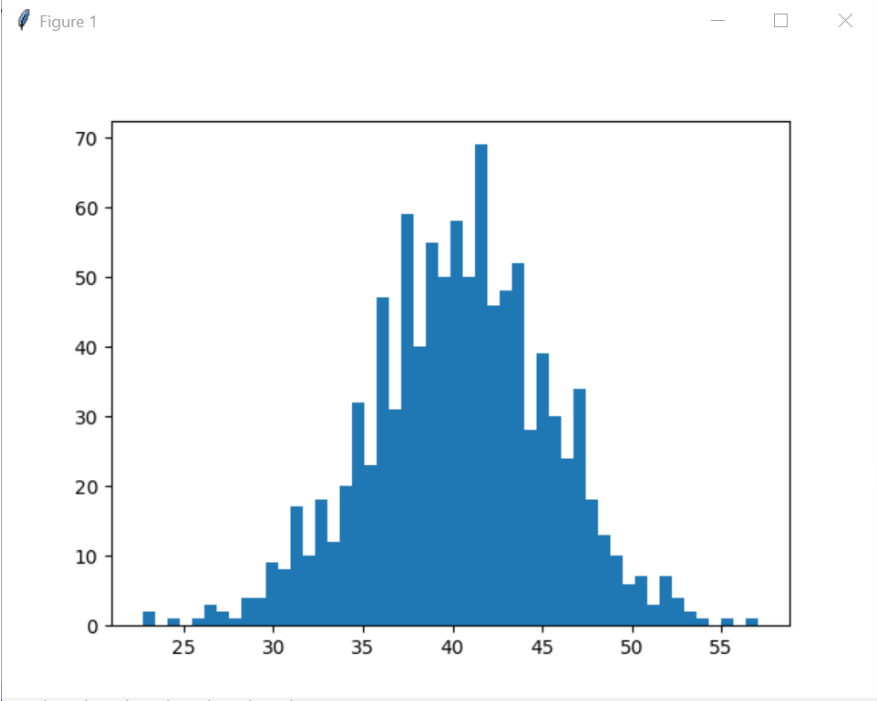## Variance

According to wikipedia , It measures how far a set of (random) numbers are spread out from their average value. In probability theory and statistics, variance is the expectation of the squared deviation of a random variable from its mean.

## Standard Deviation

According to wikipedia , In statistics, the standard deviation (SD, also represented by the lower case Greek letter sigma σ or the Latin letter s) is a measure that is used to quantify the amount of variation or dispersion of a set of data values.
A low standard deviation indicates that the data points tend to be close to the mean (also called the expected value) of the set, while a high standard deviation indicates that the data points are spread out over a wider range of values.
SD can also be treated as square root of Variance.

## Program: Standard Deviation and Variance in Python

import numpy as np
import matplotlib.pyplot as plt

#Generate ages instead of hard coding, we will get more meaningful values
#40 is centered value
#5 standard deviation
#1000 number of values
ages = np.random.normal(40, 5, 1000)
#Now ages is a list. How to get Standard deviation and variance of the list

print("Standard Deviation:", ages.std())
print("Variance:", ages.var())

Output
Standard Deviation: 5.24306013408
Variance: 27.4896795696

## Probability Density Function (PDF)

Referring to wikipedia: Probability of random variable for a speific value in continuous data is almost '0'. However, there will be a +ve value for probability of the random variabe falling within a particular range of values.
Probability Density Function is used to specify the probability of the random variable falling within a particular range of values, as opposed to taking any one value.

## Probability Mass Function (PMF)

Referring to wikipedia: This is used for set of discrete values. Probability mass function gives probability that a discrete random variable is exactly to some value.

## Draw a Uniform Distribution Curve

Program
#Draw a uniform distribution
import numpy as np
import matplotlib.pyplot as plt

#Get a list of random values and use uniform function
#start value, end value and number of points
values = np.random.uniform(-10.0, 20.0, 100000)
#Plot a histogram with 50 bars
plt.hist(values, 50)
#Show the histogram
plt.show()

Output: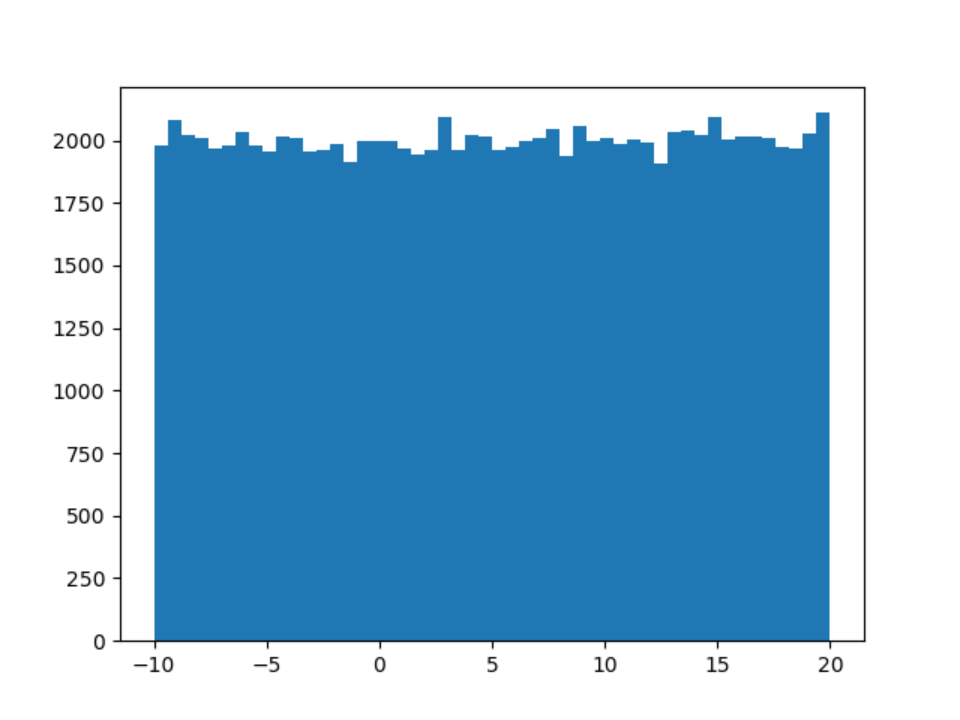## Draw a Normal Distribution Curve: using Probability Density Function

Program
from scipy.stats import norm
import matplotlib.pyplot as plt
import numpy as np

#Get random values between -5 and 5 with interval of 0.1
x = np.arange(-5, 5, 0.1)

#Use normal probability density function to get the histogram
plt.plot(x, norm.pdf(x))

#Show the histogram
plt.show()

Output: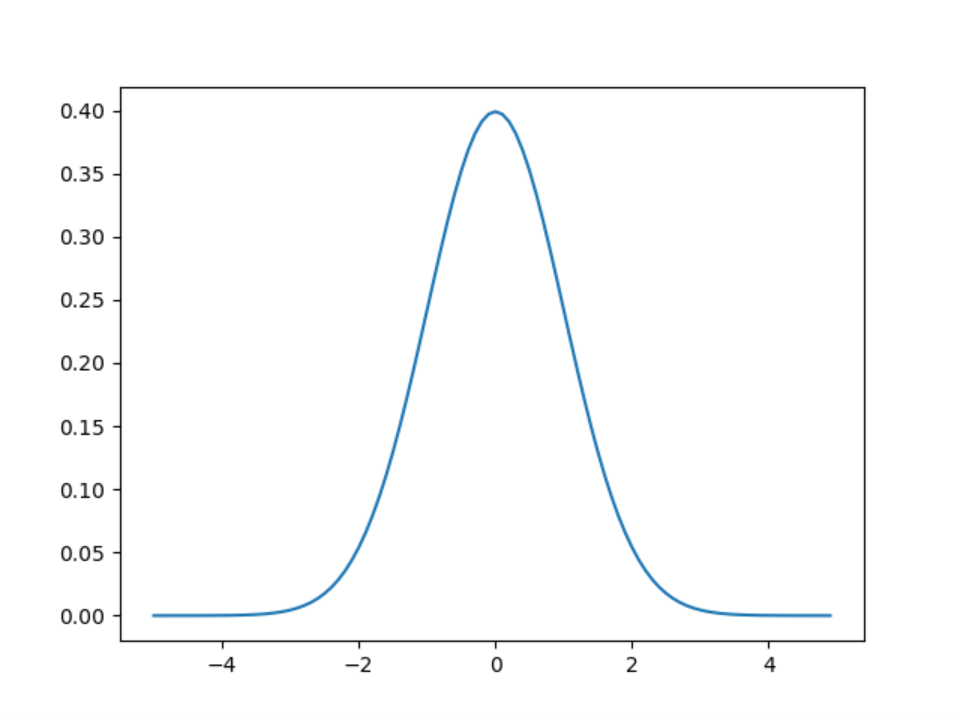## Draw a Binomial Distribution Curve: using Probability Mass Function

Program
#Binomial Distribution
from scipy.stats import binom
import matplotlib.pyplot as plt
import numpy as np

n, p = 10, 0.5
x = np.arange(0, 10, 0.001)

plt.plot(x, binom.pmf(x, n, p))
plt.show()

Output: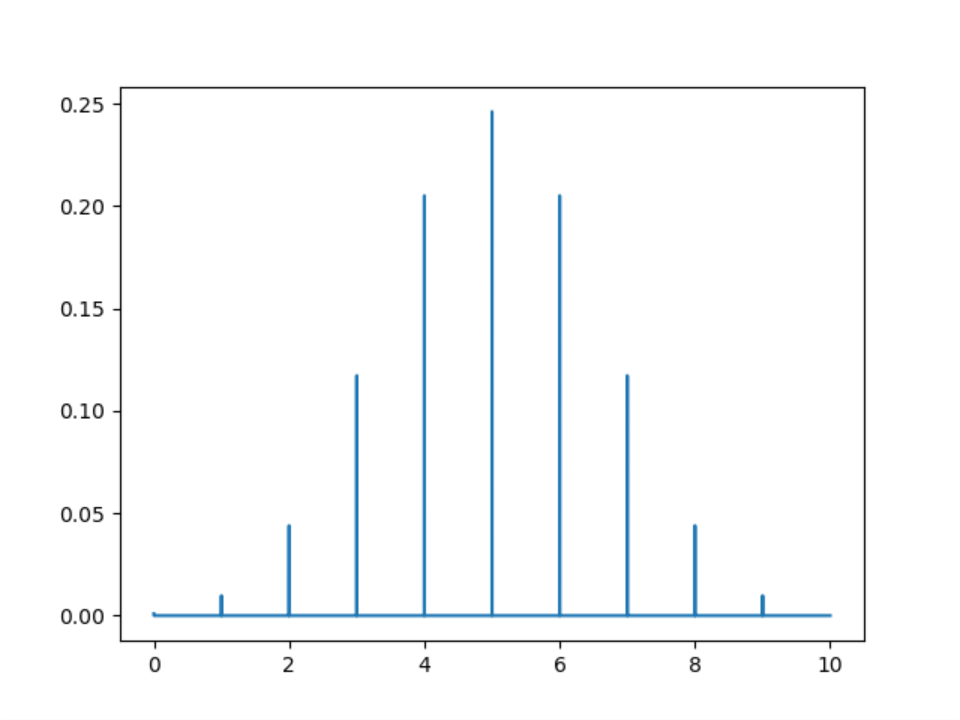## Poisson Probability Mass Function

Program
#A Restaurant gets 200 guests on average per day.
#What is the probabiity of getting 220 on a day
from scipy.stats import poisson
import matplotlib.pyplot as plt
import numpy as np

mu = 200
x = np.arange(140, 270, 0.5)
plt.plot(x, poisson.pmf(x, mu))
plt.show()

Output: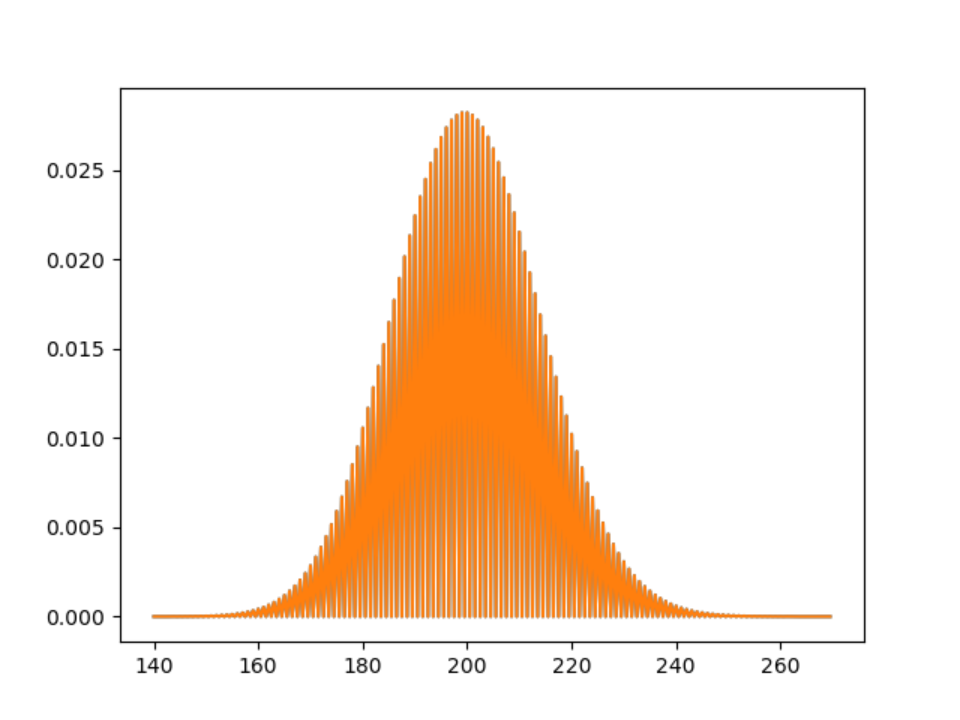## Percentile

A percentile (or a centile) is a measure used in statistics to indicate how much % is below a value.
For example: A student got 80th peercentile score in an exam means, 80% of the students got score below that sutdent.

50th percentile is equalent to Median. That is the mid value among all.

## Percentile in Python

Program
import matplotlib.pyplot as plt
import numpy as np

vals = np.random.normal(50, 4, 10000)
print ("50th percentile:", np.percentile(vals,50))
print ("10th percentile:", np.percentile(vals,10))
print ("90th percentile:", np.percentile(vals,90))

Output: (this may vary as we used random values)
50th percentile: 50.0183934715
10th percentile: 44.8104131231
90th percentile: 55.2289663083

## Moments in Statistics

1st Moment is same as Mean
2nd Momemn is Variance
3rd Moment is Skew
4th Moment is Kurtosis

Skew and Kurtosis indicate shape and sharpness of the curve of a histogram.
Skew may be -ve or +ve.
Higher the kurtosis, sharper the curve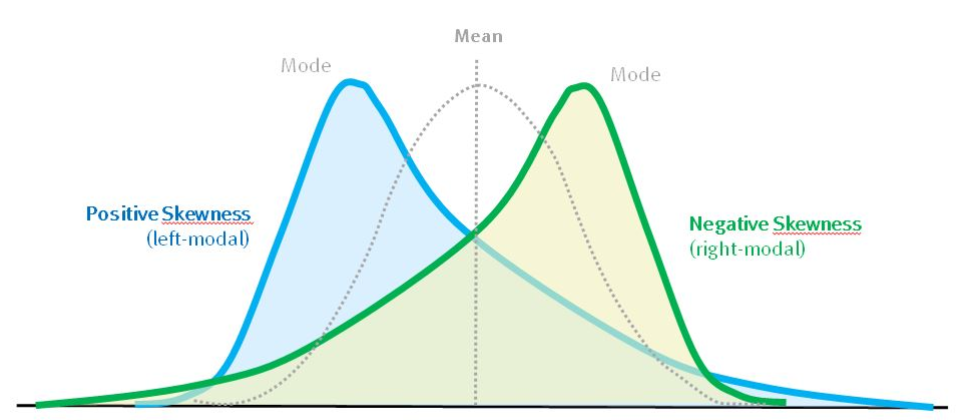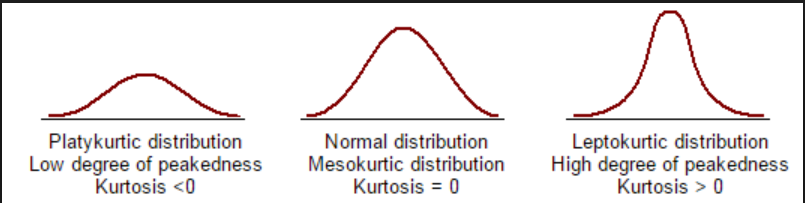### Data Science with Python and Machine Learning Reviews

Data Science with Python and Machine Learning Training: 5 Out of 5
Rated 5/5 based on 40 reviews

﻿